# ML Aggarwal Class 10 Solutions for ICSE Maths Chapter 16 Constructions Ex 16.2

## ML Aggarwal Class 10 Solutions for ICSE Maths Chapter 16 Constructions Ex 16.2

ML Aggarwal Class 10 Solutions for ICSE Maths Chapter 16 Constructions Ex 16.2

Question 1.
Draw an equilateral triangle of side 4 cm. Draw its circumcircle.
Solution: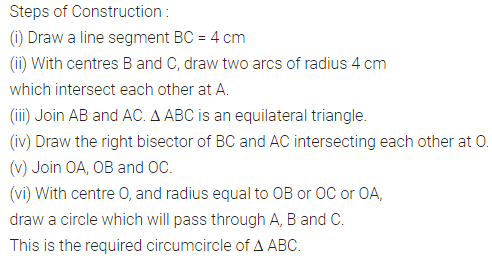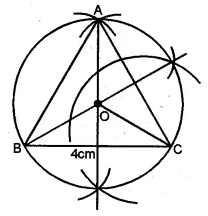Question 2.
Using a ruler and a pair of compasses only, construct:
(i) a triangle ABC given AB = 4 cm, BC = 6 cm and ∠ABC = 90°.
(ii) a circle which passes through the points A, B and C and mark its centre as O. (2008)
Solution:Question 3.
Construct a triangle with sides 3 cm, 4 cm and 5 cm. Draw its circumcircle and measure its radius.
Solution:Question 4.
Using a ruler and compasses only:
(i) Construe a triangle ABC with the following data:
Base AB = 6 cm, AC = 5.2 cm and ∠CAB = 60°.
(ii) In the same diagram, draw a circle which passes through the points A, B and C. and mark its centre O.
Solution: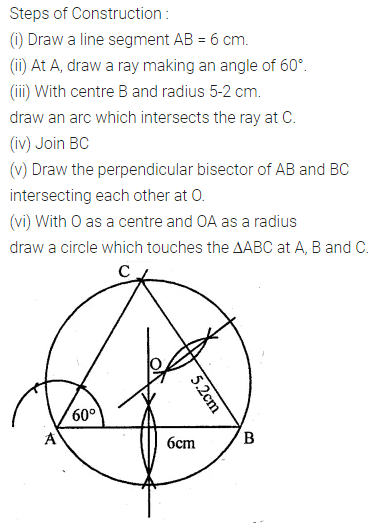Question 5.
Using ruler and compasses only, draw an equilateral triangle of side 5 cm and draw its inscribed circle. Measure the radius of the circle.
Solution:Question 6.
(i) Conduct a triangle ABC with BC = 6.4 cm, CA = 5.8 cm and ∠ ABC = 60°. Draw its incircle. Measure and record the radius of the incircle.
(ii) Construct a ∆ABC with BC = 6.5 cm, AB = 5.5 cm, AC = 5 cm. Construct the incircle of the triangle. Measure and record the radius of the incircle. (2014)
Solution: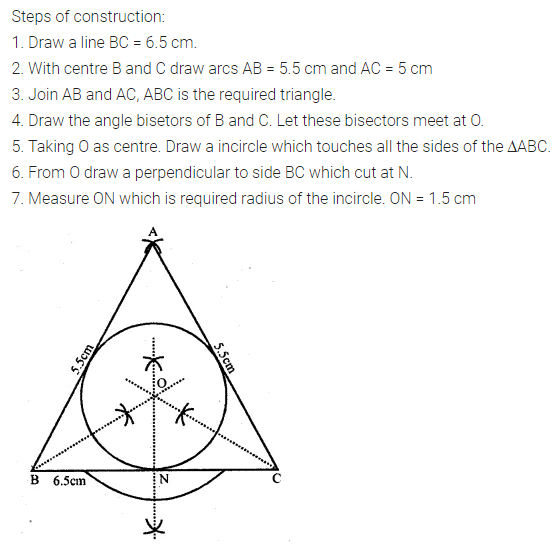Question 7.
The bisectors of angles A and B of a scalene triangle ABC meet at O.
(i) What is the point O called?
(ii) OR and OQ is drawn a perpendicular to AB and CA respectively. What is the relation between OR and OQ?
(iii) What is the relation between ∠ACO and ∠BCO?
Solution: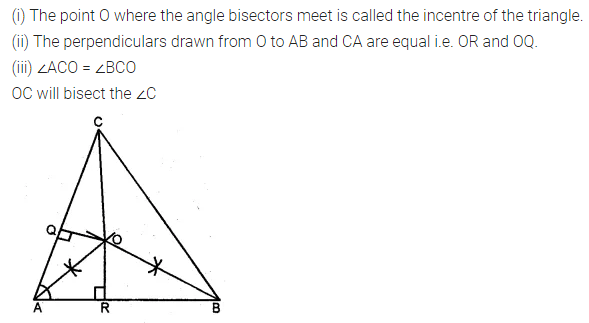Question 8.
Using ruler and compasses only, construct a triangle ABC in which BC = 4 cm, ∠ACB = 45° and the perpendicular from A on BC is 2.5 cm. Draw the circumcircle of triangle ABC and measure its radius.
Solution: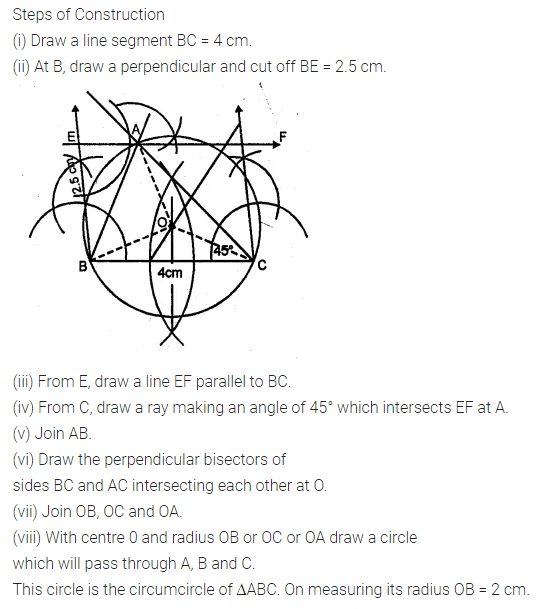Question 9.
Construct a regular hexagon of side 4 cm. Construct a circle circumscribing the hexagon.
Solution: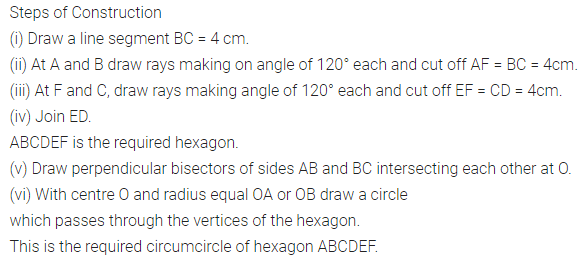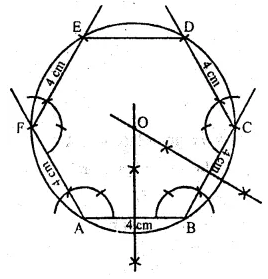Question 10.
Draw a regular hexagon of side 4 cm and construct its incircle.
Solution:ML Aggarwal Class 10 Solutions for ICSE Maths